0 results for
ICT
Computing# 3.3.1 - Understanding Number Bases

##### Overview##### Learning Objectives
IDSkill_name
3.3.1.1 I can understand decimal (base 10)
3.3.1.2 I can understand binary (base 2)
3.3.1.3 I can understand hexadecimal (base 16).

## Lesson Video

•Watch the Lesson video
Make notes on your notes sheet.
•Make notes on your notes sheet.
•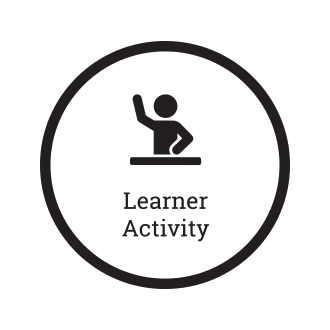Complete the learning activities
Make any notes on your notes sheet.
•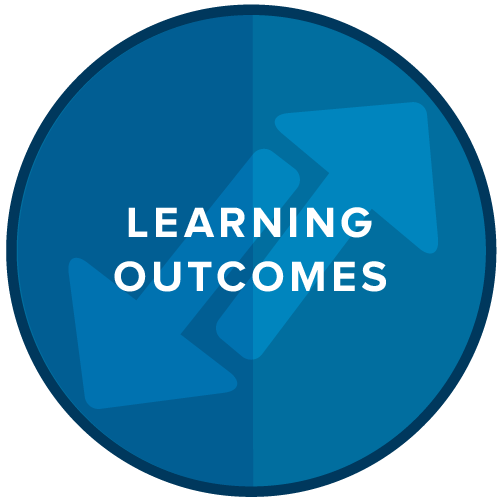Check the Learning outcomes

## Visualizing Base-10

The denary or decimal number system is the most common number system used today. It represents numbers in base 10. As you can see below each column represents a value 10 times bigger than the previous column.

So to write the number 3140 we do so like this:## Binary

Binary | Interactive | Computing

What is binary? Many people think that our counting system is based on 10s because we have ten fingers, and use them to count. Computers don't have fingers - they have electrical circuits, and electrical circuits have two states - on or off. Computers, therefore, use a number system based on twos, called binary.

In many ways, they are the same. In a number system based on tens, each column heading (units, tens, hundreds, etc.) is ten times the value of the column heading to its right, and you can use one of ten values (1-9 and 0) in each position. In a number system based on twos, each column heading is two times the one to its right, and you can use one of two values in each position.

Here you can see a binary number with the column headings added. After the equals sign is the number as we'd normally write it.

You can click each digit to toggle it between 0 and 1. If you change the binary number so that it reads 00001010, that means that you want one 8 and one 2, so the value of 00001010 is 10. It's as simple as that!

128
0
64
0
32
0
16
0
8
0
4
0
2
0
1
0
= 0

Click to investigate. Can you make 100? Is there only one pattern of 0s and 1s that make each number? A sequence of eight bits (0s or 1s), like the one shown above, is called a byte. What is the maximum number that a byte can store? If you used your 10 fingers to count in binary, you could actually count up to 1023!

## Learning Outcomes

•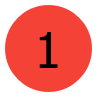I can understand what a number base is and and how
it represents numbers.

•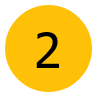I can explain what denary is and how it is different
to binary.

•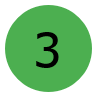I can explain what binary is and how it works as a
number system.